# Application of Deep Learning and Brain Images in Diagnosis of Alzheimer’s Patients

Application of Deep Learning and Brain Images in Diagnosis of Alzheimer’s Patients
Yueyang Vocational and Technical College, Yueyang 414000, China

Sehan University, 1113, Noksaek-ro, Samho-eup, Yeongam-gun, Jeollanam-do, 58447, Republic of Korea

Corresponding Author Email:
jy9701241@163.com
Page:
1431-1438
|
DOI:
https://doi.org/10.18280/ts.380518
29 May 2021
|
Accepted:
1 September 2021
|
Published:
31 October 2021
| Citation

OPEN ACCESS

Abstract:

In the identification of which stages Alzheimer’s patients are in, the application of the medical imaging technology helps doctors give more accurate qualitative diagnoses. However, the existing research results are not effective enough in the acquisition of valuable information from medical images, nor can they make full use of other modal images that highlight different feature information. To this end, this paper studies the application of deep learning and brain images in the diagnosis of Alzheimer’s patients. First, the image preprocessing operations and the brain image registration process were explained in detail. Then, the image block generation process was given, and the degrees of membership to white matter, gray matter and cerebrospinal fluid were calculated, and the brain images were also preliminarily classified. Finally, a complete auxiliary diagnosis process for Alzheimer’s disease based on deep learning was provided, an improved sparse noise reduction auto-encoder network was constructed, and the brain image recognition and classification based on deep learning were completed. The experimental results verified the effectiveness of the constructed model.

Keywords:

deep learning, brain image recognition, Alzheimer’s disease

1. Introduction

With the aging of population, the prevalence of Alzheimer’s disease is increasing year by year [1-3]. While the cause of Alzheimer’s disease is still uncertain, it can bring various irreversible harms to patients such as loss of memory, mobility impairment and loss of cognitive function [4-7]. Fortunately, the rapidly developing medical imaging technology, which provides abundant high-quality ecological and physiological information [8-11], can be applied to identify which stages Alzheimer’s patients are in, and in this way, doctors can give more accurate qualitative diagnosis of patients’ conditions. Now in the era of big data, there have been increasingly more deep learning methods applicable for large-scale, high-dimensional medical imaging analysis [12-14]. And therefore, how to improve a neural network model’s ability to analyze medical images of Alzheimer’s disease has become a realistic and also challenging task.

Early diagnosis plays an important role in the prevention and treatment of Alzheimer’s disease. Moeskops et al.  proposed predicting Alzheimer’s disease with a deep three-dimensional convolutional neural network. The network can learn and capture the general features of Alzheimer’s biomarkers and adapt to datasets in different fields. Hosseini-Asl et al.  emphasized the inaccuracy and limitations of some Alzheimer’s disease test methods such as clinical history examination, simple mental status examination and paired associate learning, and suggested that the more accurate diagnosis method based on neuroimaging data would be the future trend. Gunawardena et al.  successfully distinguished the functional magnetic resonance imaging (MRI) data of Alzheimer’s patients from those of the normal control group using the convolutional neural network and the famous LeNet-5 structure, with an accuracy of 96.85%. Sarraf, and Tofighi  pointed out that Alzheimer’s disease may be partially caused by the loss of white matter integrity and interruption of connectivity and that the new microstructure measurement values derived from the additional dMRI model may contain the geometric structure, diffusion rate and complexity of diffusion anisotropy, the estimated number of distinguishable fiber compartments, the number of decussating fibers, and the dispersion of nerve axons. Nir et al. proposed an improved hippocampus segmentation method based on the watershed algorithm, and used two methods to convert brain images to the binary form - the first one is block averaging, mask and concept tagging, and the second is top hat, mask and concept tagging [19, 20]. Ismail et al.  conducted a comparative study on the four types of fractional-order filters for edge detection, analyzed the noise performance of these filters under random Gaussian noise and salt-and-pepper noise, and gave a numeric comparison of the peak signal-to-noise ratios of the detected images.

Through comparison of the studies on different medical image classification methods, it can be seen that, in previous studies, most experiments required a lot of work to preprocess single-modal medical images and extract image features based on priori knowledge, which not only reduces the valuable information acquired from medical images, but also makes it impossible to make full use of other modal images that highlight different feature information. To this end, this paper studies the application of deep learning and brain images in the diagnosis of Alzheimer’s patients. First, Section 2 elaborates on the preprocessing operations such as deskulling, template generation, template segmentation and reconstruction, template registration and grayscale normalization of images, and introduces in detail the brain image registration process. Section 3 gives the image block generation process, and completes the calculation of the membership degrees of brain tissues, namely white matter, gray matter and cerebrospinal fluid, and the preliminary classification of brain images. Section 4 provides a complete auxiliary diagnosis process for Alzheimer’s disease based on deep learning, constructs an improved sparse noise reduction auto-encoder network, and completes brain image recognition based on deep learning. The experimental results prove the effectiveness of the constructed model.

2. Brain Image Preprocessing Method

In this section, 100 samples of structural MRI images and positron emission tomography (PET) images of the same individual were first selected from the ADNI database, which is dedicated to research on reducing or suppressing the progression of Alzheimer’s disease, and then, all the sample images were pre-processed through a number of operations, including deskulling, template generation, template segmentation and reconstruction, template registration, and image grayscale normalization. The registration process of the structural MRI images and PET images of the Alzheimer’s patient is described in detail below. Figure 1 shows the corresponding brain image preprocessing process.

## 1.png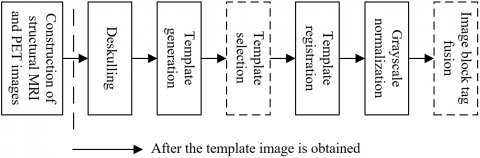Figure 1. Preprocessing process of the brain images of an Alzheimer’s patient

The registration of structural MRI images and PET images is a spatial conversion process mapping the points on the reference template image to the homologous points on the image to be registered. Figure 2 shows the registration process of the brain images of the Alzheimer’s patient. In this paper, the mutual information method was used to estimate the registration quality of the image to be registered and the reference image, which is to obtain the mutual information as the multi-modal medical image registration criterion based on the calculation of the generalized distance between the joint probability distribution and the independent probability distribution of the image to be registered and the reference one.

## 2.png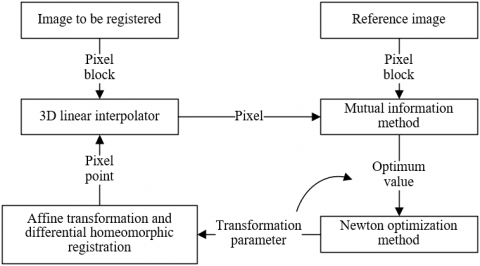Figure 2. Registration process of brain images

Given two 3D images A and B after transformation o, assuming that the gray values of the points on images A and B are represented by a and b, respectively, that the joint grayscale histogram of images A and B is denoted as fo(a,b), and that the corresponding independent and joint probability density functions are represented by ρA,o(a), ρB,o(b) and ρAB,o(a,b), then:

$\rho_{A, o}(a, b)=\sum_{b} \rho_{A B, o}(a, b)$         (1)

$\rho_{B, o}(a, b)=\sum_{a} \rho_{X Y, o}(a, b)$         (2)

$\rho_{A B, o}(a, b)=\frac{f_{o}(a, b)}{\sum_{a, b} f_{o}(a, b)}$         (3)

Eq. (4) gives the definition of mutual information HX(β):

$H X(\beta)=\sum_{a, b} \rho_{A B, \beta}\quad(a, b) \log _{2} \frac{\rho_{A B, \quad\beta}\quad(a, b)}{\rho_{A, \beta}\quad(a) \rho_{B, \beta}\quad(b)}$         (4)

The optimal registration parameter β* can be calculated by Eq. (5):

$\beta^{*}=\max _{\beta} H X(\beta)$         (5)

Finding β* based on the search optimization algorithm is the registration process of images A and B, whose purpose is to maximize HX(β).

During the registration process, it is necessary to compare the brightness of the image to be registered with that of the reference image. An interpolator can be used to calculate the brightness of a point on the reference image that is mapped to a non-grid location on the image to be registered. Since the brightness values of the 8 vertices of the cubic grid unit where the point is located on the reference image are known, a trilinear interpolator that can perform 3D linear interpolation on 8 brightness values was applied here. Suppose that the brightness values of the 8 points are represented by Q0-Q7, respectively. According to the definition of trilinear interpolation, the pixel value UQ of the point Q(a,b,c) on the reference image can be expressed by Eq. (6):

\begin{aligned} &B_{Q}=Q_{0}(1-a)(1-b)(1-c)+Q_{1}(1-a) b(1-c) \\ &+Q_{2}(1-a)(1-b) c+Q_{3}(1-a) b c \\ &+Q_{4} a(1-b)(1-c)+Q_{5} a b(1-c)+Q_{6} a(1-b) c+Q_{7} a b c \end{aligned}         (6)

The optimizer selected in this paper uses the idea of Newton’s method, as shown in Eq. (7):

$a^{0} \Rightarrow a^{1} \Rightarrow \ldots \Rightarrow a^{l} \Rightarrow a^{l+1} \Rightarrow \ldots$         (7)

In order to generate al+1 from al, the quadratic function W(a) is used to approximate g(a), and then there is:

\begin{aligned} & g(a) \approx W(a)=g\left(a^{l}\right)+\nabla g\left(a^{l}\right)^{T}\left(a-a^{l}\right) \\ &+\frac{1}{2}\left(a-a^{l}\right)^{T} \nabla^{2} g\left(a^{l}\right)\left(a-a^{l}\right) \\ =& g\left(a^{l}\right)+h_{l}^{T} \cdot\left(a-a^{l}\right)+\frac{1}{2}\left(a-a^{l}\right)^{T} H_{l}\left(a-a^{l}\right) \end{aligned}         (8)

where, hl=∇g(al)T, Hl=∇2g(al). Let ∇W(a)=hl+Hl(a-al)=0. If the Hesse matrix Hl is positive definite, that is, Hl>0, then Hl-1>0, and there is Newton’s iterative formula:

$a^{l+1}=a^{l}-H_{l}^{-1} h_{l}$         (9)

Affine transformation is a global transformation in linear image registration. The purpose is to make the images to be registered at different time, from different imaging devices and under different conditions spatially consistent with the reference image.

Suppose that there are two 3D images P1 and P2, among which, the image to be registered is denoted as P1, and the reference image as P2, and that their corresponding gray values are denoted as P1(a,b,c) and P2(a,b,c). Suppose that the 3D spatial geometric transformation is represented by g, and that the 1D grayscale transformation by h. Eq. (10) shows the registration process of P1 and P2:

$P_{2}(a, b, c)=h\left(P_{1}(g(a, b, c))\right)$         (10)

Spatial transformation and geometric transformation are the keys to the registration of the two images, and the grayscale transformation h is generally unnecessary. Based on this, the above formula can be converted to:

$P_{2}(a, b, c)=P_{1}(g(a, b, c))$         (11)

There are four types of geometric transformations in linear registration - translation, scaling, cropping and rotation, which are not elaborated in detail here. Instead, the Log-Domain differential homeomorphic registration method for local registration is described in detail. This method can realize the registration of the details of the image to be registered, that is, it can find the most suitable spatial transform r that realizes the optimal matching of the images P0 and P1.

Assuming that the similarity of two images can be characterized by the similarity criterion Com(P0,P1,r), and that the normalization term used to control the smoothness of r is represented by NO h(r), the following energy equation can be established:

$S(r)=\frac{1}{\phi_{i}^{2}} \operatorname{Com}\left(P_{0}, P_{1}, r\right)+\frac{1}{\phi_{N O}^{2}} N O h(r)$         (12)

where, the parameters φi and φNO are respectively used to control the noise in the image and the degree of normalization required for registration. The deformation parameters need to be continuously optimized during the registration process. Assuming that the unit transformation during algorithm initialization is represented by r, and that the update field calculated in each iteration in the optimization and iteration process of the deformation parameters is represented by λ, then:

$S_{\text {add }}^{\text {corr }}\left(P_{0}, P_{1}, r, \lambda\right)=\operatorname{Com}\left(P_{0}, P_{1}, r+\lambda\right)+\|\lambda\|^{2}$         (13)

where, λ is added to r at the end of each iteration; in other words, r will be updated every time the iteration is completed until the end of the iteration.

If the optimization process is constrained within the differential homeomorphic space, deformation fields that meet the continuous, smooth, and one-to-one mapping requirements can be obtained. All the deformation fields form a Lie group, and then the updated energy equation in the differential homeomorphic space can be further obtained, as shown in Eq. (14):

$S_{\text {diffeo }}^{\text {corr }}\left(P_{0}, P_{1}, r, \lambda\right)=\operatorname{Com}\left(P_{0}, P_{1}, r \circ \exp (\lambda)\right)+\|\lambda\|^{2}$         (14)

where, the “○” operation is a combination of spatial transformation + superposition, which is more accurate and reasonable than direct superposition of quantitative values.

In order to simplify the calculation process, the entire differential homeomorphic mapping is optimized in the logarithmic domain, so here eu is used to take the place of r, and then:

$e^{d}=r \circ e^{\lambda}=e^{u} \circ e^{\lambda}$         (15)

The Log-Domain differential homeomorphic optimization process is described in detail as follows:

Step1: Determine the initial value as follows:

$\psi=P t, \psi^{\prime}=P t$         (16)

Step2: Complete the first iteration of the optimization process based on the following formula:

$\begin{gathered} v^{g}=\frac{P_{0}-P_{1} \circ \psi^{(1)}}{L_{1}} \cdot L_{2}=\frac{P_{0}-P_{1}}{L_{1}} \cdot L_{2} \\ v^{y}=\frac{P_{1}-P_{0} \circ \psi^{\prime}}{L_{1}} \cdot L_{2}=\frac{P_{0}-P_{1}}{L_{1}} \cdot L_{2} \end{gathered}$         (17)

where,

$L_{1}=\left\|I^{q}\right\|^{2}+\frac{\phi_{i}^{2}(q)}{\phi_{a}^{2}}, L_{2}=I^{q \gamma}, I^{q \gamma}=-\nabla_{q}^{\gamma}(N \circ r)$         (18)

That is, perform total differentiation in all directions in the differential homeomorphic space:

\begin{aligned} &u^{(1)}=\tilde{C}^{(2)}=\frac{1}{2}\left(C+C^{\prime}\right) \\ &=\frac{1}{2}\left[\left(u^{(0)}+\lambda^{g(1)}\right)+\left(u^{(0)}+\lambda^{y(1)}\right)\right] \\ &=\frac{1}{2}\left[\log (P t)+\lambda^{g(1)}+\log (P t)+\lambda^{y(1)}\right] \end{aligned}         (19)

$\psi^{(1)}=\exp \left(u^{(1)}\right), \psi^{\prime(1)}=\exp \left(-u^{\prime(1)}\right)$         (20)

Step3: Complete the second iteration of the optimization process based on the following formula:

$v^{g(2)}=\frac{P_{0}-P_{1} \circ \psi^{(1)}}{L_{1}} \cdot L_{2}, v^{v(2)}=\frac{P_{1}-P_{0} \circ \psi^{(1)}}{L_{1}} \cdot L_{2}$           (21)

\begin{aligned} &u^{(2)}=\tilde{C}^{(2)}=\frac{1}{2}\left(C^{(2)}+C^{(2)}\right) \\ &=\frac{1}{2}\left[\left(u^{(1)}+\lambda^{g(2)}\right)+\left(u^{\prime(1)}+\lambda^{y(2)}\right)\right] \\ &=\frac{1}{2}\left[u^{(1)}+\lambda^{g(2)}+\left(-u^{(1)}+\lambda^{y(2)}\right)\right] \end{aligned}           (22)

$\psi^{(2)}=\exp \left(u^{(2)}\right), \psi^{\prime(2)}=\exp \left(-u^{\prime(2)}\right)$           (23)

Step3: Repeat the operation to obtain the optimal result:

$\psi^{(m)}=\exp \left(u^{(m)}\right), \psi^{\prime(m)}=\exp \left(-u^{(m)}\right)$           (24)

3. Image Block Tag Fusion

## 3.png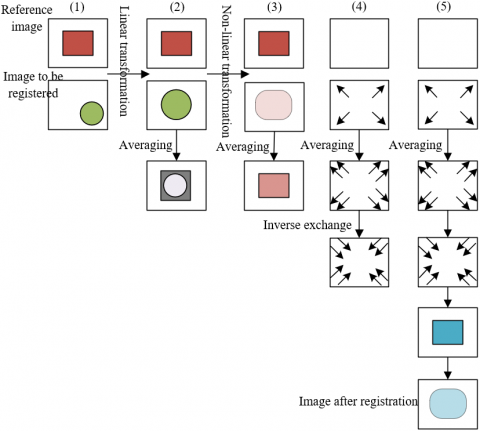Figure 3. Image block generation process

As an algorithm for local weighted voting, the tag fusion algorithm based on the similarity of image blocks believes that the more similar a pixel point or image block of the image to be registered is with that of the reference image, the greater weight the corresponding tag has. Figure 3 shows the schematic diagram of the image block generation process. The similarity of image blocks is often measured by Euclidean distance. First, analyze the scenario where the image block is extremely small, that is, there is only one pixel point in the block. Assuming that the image block of the image to be registered is represented by TU1=[h1], and that the image block of the reference image is represented by TU2=[h2], Eq. (25) gives the calculation formula of the Euclidean distance D between the two:

$\delta=h_{1}-h_{2}$           (25)

It can be seen from the above formula that the Euclidean distance between the pixel of the image to be registered and that of the reference image is the difference between the two pixel points. If the pixel values of the two pixels are equal, that is, TU1=TU2, then the similarity of the images can be expressed as δ=0. For an actual image with noise, when δ is not equal to 0, the pixel values of the two pixels may still be equal. In this paper, the Gaussian-weighted Euclidean distance shown in Eq. (26) was used to measure the similarity between the two:

$H=e^{-\frac{\left(h_{1}-h_{2}\right)^{2}}{f}}$         (26)

When the image block contains m pixels, let TU1-=[h1,h2,…,hn ]T and TU2-=[h'1,h'2,…,h'm]T, and then the calculation formula of the Euclidean distance between the two is expressed as Eq. (27):

$\delta=\left\|\overline{T U_{1}}-\overline{T U_{2}}\right\|_{2}^{2}$          (27)

Assuming that the 2-norm of the vector is represented by ||*||2, then:

\begin{aligned} &\left\|\overline{T U_{1}}-\overline{T U_{2}}\right\|_{2} \\ &=\sqrt{\left(h_{1}-h_{1}^{\prime}\right)^{2}+\left(h_{2}-h_{2}^{\prime}\right)^{2}+\ldots+\left(h_{m}-h_{m}^{\prime}\right)^{2}} \end{aligned}          (28)

Eq. (29) shows the corresponding Gaussian weighted Euclidean distance calculation formula:

$H=e^{-\frac{\left|\overline{T U_{1}}-\overline{T_{2}}_{2}\right|\quad_{2}^{2}}{f}}$          (29)

For the pixel i of the image to be registered, the image block TU with a size of m×m and 1 as the center pixel is represented by a column vector with a size of m2×1. Suppose that the image blocks in the similar set of TU is denoted as SIBj, and that the number of image blocks in the similar set as n, then j[0,n]. The similar set S={SIBj} of TU is an m2×n matrix. The weight χ={χj} of the image blocks in the similar set is an n×k column vector. Assuming that the parameter that controls the sparseness of the weight coefficients is denoted as σ, the sparse decomposition formula based on image blocks is expressed as follows:

$\chi^{*}=\arg \min _{\chi} \frac{1}{2}\|S I B-S \chi\|^{2}+\sigma\|\chi\|_{1}$          (30)

where, χ* is the sparse weight of the image blocks in the similar set. After χ* is obtained, the tag values of the image blocks in the image to be registered can be further obtained based on local weighted voting. Instead of binary local weighted voting, the goal of this research is to achieve the ternary classification of brain tissues in the structural MRI images and PET images, which requires comparison of the degrees of membership to different brain tissues.

Suppose that the three column vectors used to characterize whether the pixel point at the corresponding position of TUj belongs to any of the three types of brain tissues - white matter, gray matter, and cerebrospinal fluid - are represented by Konj, Khnj and Kzrgj, and that the size of each column vector is m2×1. Suppose that the column vectors characterizing the corresponding degree of membership is represented by Uoni, Uhni and Uzrg-i, and that the size is also m2×1. The tag fusion algorithm based on the local weighted voting mechanism is described in detail as follows:

First, for any pixel point i of the brain region Ψ, calculate the degrees of its membership to the three types of brain tissues - white matter, gray matter, and cerebrospinal fluid – according to Eq. (31), (32) and (33) as follows:

$U^{h n}=\frac{\sum_{j=1}^{n} \chi_{j} \cdot K_{j}^{h n}}{\sum_{j=1}^{n} \chi_{j}}$          (31)

$U^{o n}=\frac{\sum_{j=1}^{n} \chi_{j} \cdot K_{j}^{o n}}{\sum_{j=1}^{n} \chi_{j}}$          (32)

$U^{z r g}=\frac{\sum_{j=1}^{n} \chi_{j} \cdot K_{j}^{z r g}}{\sum_{j=1}^{n} \chi_{j}}$          (33)

Then compare the degrees of membership, that is, Uoni, Uhni and Uzrg-i, of the same pixel point, and classify the pixel point to the type with the largest membership degree. Finally, repeat the above two steps until all pixels are classified.

4. Brain Image Recognition Based on Deep Learning

## 4.png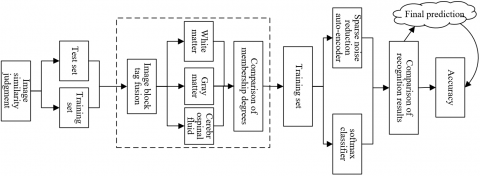Figure 4. Auxiliary diagnosis process for Alzheimer’s disease based on deep learning

In order to obtain more accurate recognition results of structural MRI and PET images, an improved sparse noise reduction auto-encoder network was constructed, which contains a sparse noise reduction auto-encoder and a softmax classifier, and the quasi-Newton method was used to solve the cost function of sparse noise reduction auto-encoding. Figure 4 shows a complete auxiliary diagnosis process for Alzheimer’s disease based on deep learning. Figure 5 shows the structure of the proposed improved sparse noise reduction auto-encoder network.

Assuming that the step size is represented by ξl, and that the direction of the l-th search is represented by FXl, the iterative formula of the quasi-Newton method is expressed by Eq. (34):

$a_{l+1}=a_{l}+\xi_{l} F X_{l}$          (34)

The step size ξl can be calculated by Eq. (35):

$\xi_{l}=\operatorname{argmin} g\left(a_{l}+\xi_{l} F X_{l}\right)$          (35)

Suppose that the inverse matrix of the Hessian matrix is represented by NJl, and that the second-order differentiable objective function is represented by g, the direction FXl of the l-th search can be calculated by Eq. (36):

$F X_{l}=-N J_{l} \nabla g_{l}$          (36)

In order to solve the storage problem of the approximate Hessian matrix, the quasi-Newton method based on limited memory was adopted in this paper, which uses the gradient information of the last n iterations to construct NJl on the basis of the original algorithm. Eq. (37) shows the update formula of NJl:

\begin{aligned} &N J_{l}=\left(U_{l-1}^{T} \ldots U_{l-n}^{T}\right) N J_{l}^{0}\left(U_{l-n} \ldots U_{l-1}\right) \\ &+\left(U_{l-1}^{T} \ldots U_{l-n+1}^{T}\right) \varepsilon_{l-n} C H_{l-n} C H_{l-n}^{T}\left(U_{l-n+1} \ldots U_{l-1}\right) \\ &+\left(U_{l-1}^{T} \ldots U_{l-n+2}^{T}\right) \varepsilon_{l-n} C H_{l-n+1} C H_{l-n+1}^{T}\left(U_{l-n+2} \ldots U_{l-1}\right) \\ &+\ldots \\ &+\varepsilon_{l-1} C H_{l-1} C H_{l-1}^{T} \end{aligned}          (37)

where,

$U_{l}=S R-\varepsilon_{l} C F_{l} C H_{l}^{T}$          (38)

$\varepsilon_{l}=\frac{1}{C F_{l}^{T} C H_{l}}$          (39)

$C F_{l}=\nabla g\left(a_{l+1}\right)-\nabla g\left(a_{l}\right)$          (40)

$C H_{l}=a_{l+1}-a_{l}$          (41)

In the quasi-Newton method based on limited memory, the inverse matrix NJl of the complete Hessian matrix is no longer saved; instead, saving the vectors of the last n steps in the vector sequence CHl and CFl will suffice.

## 5.png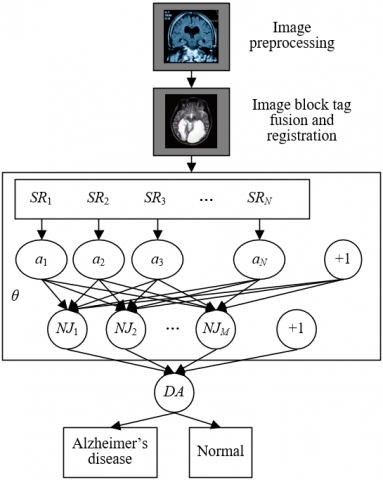Figure 5. Structure of the proposed improved sparse noise reduction auto-encoder network

In order to avoid the network over-fitting problem caused by the small sample size, the sparse penalty term EDIL and the weight of the hidden layer θ were calculated. Assuming that the weight attenuation term to prevent over-fitting is represented by EDθ, the overall cost function is expressed by Eq. (42):

$E D_{I L}=E D_{Q}+\mu E D_{\theta}+\alpha E D_{S P}$          (42)

The calculation formula of EDθ is shown in Eq. (43):

$E D_{\theta}=\frac{1}{2} \sum_{i=1}^{m} \sum_{j=1}^{F X}\left(\theta_{j i}\right)^{2}$          (43)

Assuming that the number of iterations is denoted as l, that the step size of the hidden layer as ξl, and that the vector direction as FXl, the update of EDIL and θ can be completed through Eq. (44) and (45):

$\theta_{k+1}=\theta_{k}+\xi_{l} F X_{l}$          (44)

$E D_{I L}\left(\theta_{l}+\xi_{l} F X_{l}\right)=\min E D_{I L}\left(\theta_{l}+\xi_{l} F X_{l}\right)$          (45)

Suppose that the network input vector is denoted as SR, that the weight difference of the last two iterations as DAl, and that the difference between the partial derivatives of two adjacent iterations as PDl. Through multiple experiments, NJl-n and Φl in the model can be calculated as follows:

$N J_{l}^{0}=\Phi_{l} S R$          (46)

$\Phi_{l}=\frac{D A\quad_{l-1}^{T}\quad P D_\quad{l-1}}{P D\quad_{l-1}^{T}\quad P D_\quad{l-1}}$          (47)

Through continuous repeat of the above iteration steps, the value of EDIL can be gradually reduced until the training of the neural network is completed. Finally, the updated values of EDIL and θ can be used as the input to the Softmax classifier to achieve image recognition and classification.

5. Experimental Results and Analysis

In this paper, the images to be registered for the auxiliary diagnosis after tag fusion were evaluated in terms of information entropy, correlation coefficient and peak signal-to-noise ratio. Table 1 shows the evaluation results. It can be seen that the information entropy of the tag fused brain images was improved, indicating that the amount of information contained in the images to be registered increased after the image block tag fusion. Correlation coefficient 1 (with the structural MRI image) and correlation coefficient 2 (with the PET image), peak signal-to-noise ratio 1 (to the structural MRI image), and peak signal-to-noise ratio 2 (to the PET image) were all improved. Both correlation coefficient 1 and correlation coefficient 2 increased, indicating that the images to be registered after image block tag fusion are closely correlated to the original single-modal brain images, thus verifying the feasibility of the image block tag fusion method proposed in this paper. Both peak signal-to-noise ratio 1 and peak signal-to-noise ratio 2 are large, showing that the tag fused images obtained from the proposed algorithm extracted a lot of information from the original brain images and that the denoising effect was excellent. At the same time, it can be seen that, through image block tag fusion, the registration results were good, proving that the proposed algorithm has a certain robustness.

Table 1. Evaluation of tag-fused images

 Image 0.2MRI+0.8PET 0.3MRI+0.7PET 0.4MEI+0.6PET 0.5MRI+0.5PET 0.4MRI+0.6Temp Information entropy 4.0521 4.023 4.0356 4.0532 3.8645 Correlation coefficient 1 - 0.89475 0.92574 0.98564 0.97468 Correlation coefficient 2 - - - 0.98564 0.85962 Peak signal to noise ratio 1 - - - 15.2677 16.7548 Peak signal to noise ratio 2 - - - 26.4852 24.2684 Image 0.5MRI+0.5Temp 0.6MRI+0.4Temp 0.4PET+0.6Temp 0.5PET+0.5Temp 0.6PET+0.4Temp Information entropy 3.8956 3.7648 4.0859 4.0326 4.0516 Correlation coefficient 1 - - 0.98567 0.96254 - Correlation coefficient 2 0.99647 0.98745 - - 0.96325 Peak signal to noise ratio 1 18.2574 19.2684 32.5746 34.5186 - Peak signal to noise ratio 2 22.5486 19.2643 - 18.2635 19.4856

## 6-1.png## 6-2.png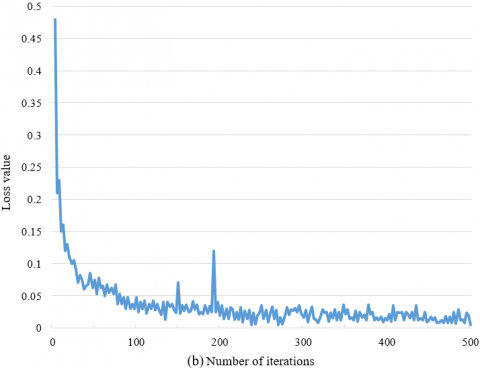Figure 6. Decline curve of the network loss function

For the ADNI database, after the registration of the brain images to be registered, the Dice values of the brain tissues, namely white matter, gray matter and cerebrospinal fluid, are higher than those obtained by the traditional image registration method. It can be seen from the comparison of the loss curves in Figure 6 that, the loss function value of the algorithm using the idea of image block tag fusion converged faster in the network training stage, and that the downward trend of the curve was more stable.

## 7.pngFigure 7. Comparison of the recognition rates of different brain tissues

Table 2 compares the brain image recognition accuracy of different models. It can be seen that method 1 proposed in this paper is better than the stacked auto-encoding + BP neural network model 2, the traditional stacked auto-encoding model 3 and the weighted multi-modal classification model 4. Then, the grayscale image features of different brain tissues were extracted, and the degrees of membership to the brain tissues - white matter, gray matter and cerebrospinal fluid - were compared based on the data source. Figure 7 shows the comparison results of the recognition rates for different brain tissues. It can be seen that the calculation method proposed in this paper is also superior to other models in terms of the brain tissue recognition rate.

Table 2. Comparison of the brain image recognition accuracy of different models

 Model No. 1 2 3 4 Training times 1 92.31 81.46 77.58 66.75 2 90.44 82.45 76.25 76.48 3 94.61 80.48 73.56 68.51 4 89.39 81.74 74.81 65.15 5 88.26 81.49 76.85 69.28 6 92.87 80.52 74.28 65.43 Mean 91.31 81.75 75.48 68.75

In order to further verify the effectiveness of the proposed algorithm in the auxiliary diagnosis of Alzheimer’s disease, the brain images of patients with mild cognitive impairment were also used in the comparative experiment to show a more comprehensive comparison of the recognition rates for different types of brain images and test the sensitivity and specificity of different models towards different image modalities. Therefore, in addition to the samples of patients from the ADNI database, 122 brain images of patients with mild cognitive impairment were also added. Table 3 shows the results of the comparative experiment on different types of images.

As can be seen from the table, in the “Alzheimer’s disease-normal” classification experiment, for MRI images, the proposed model had the highest accuracy - the accuracy of brain image recognition and classification was 91.2%, the sensitivity 92.5%, and the specificity 93.2%. In the “Alzheimer’s disease-mild cognitive impairment” classification experiment, for MRI images, the proposed model still had the highest accuracy of brain image recognition and classification - 89.2%. The specificity was 88.1%, and the sensitivity was relatively lower - 78.2%, which was lower than the sensitivity of 79.2% towards PET images.

Table 3. Experimental results of different types of images

 Type Alzheimer’s disease - normal Model No. 1 2 3 Image modality Structural MRI images PET images Structural MRI images PET images Structural MRI images PET images Accuracy 91.2 86.2 88.4 87.2 87.1 86.2 Specificity 93.2 94.3 86.2 85.1 86.2 81.2 Sensitivity 92.5 82.1 90.2 90.3 83.2 78.4 Type Alzheimer’s disease - mild cognitive impairment Model No. 1 2 3 Image modality Structural MRI images PET images Structural MRI images PET images Structural MRI images PET images Accuracy 89.2 80.2 71.4 75.2 76.4 71.0 Specificity 88.1 89.5 75.1 72.3 79.2 76.1 Sensitivity 78.2 79.2 66.4 69.2 75.2 76.8
6. Conclusion

This paper studied the application of deep learning and brain images in the diagnosis of Alzheimer’s patients. It first completed the image preprocessing operations and brain image registration. Then, it gave the image block generation process, and completed the calculation of the membership degrees of white matter, gray matter and cerebrospinal fluid. Finally, it provided a complete auxiliary diagnosis process for Alzheimer’s disease based on deep learning and constructed an improved sparse noise reduction auto-encoder network for brain image recognition and classification. The experimental results showed the evaluation on the tag fused images, and verified that the registration results of images was all good after image block tag fusion, so the proposed algorithm has certain robustness. The decline curves of the network loss function drawn verified the convergence of the proposed neural network during the training stage. The comparison of the recognition rates for different types of brain tissues and the accuracy of different models in brain image recognition proved that the proposed method is superior to other models in terms of brain tissue recognition rate. The comparative experimental results with respect to different types of images verified that the proposed model is still effective for the classification of Alzheimer’s disease - mild cognitive impairment.

References

 Kundaram, S.S., Pathak, K.C. (2021). Deep learning-based Alzheimer disease detection. Proceedings of the Fourth International Conference on Microelectronics, Computing and Communication Systems, Ranchi, India, pp. 587-597. https://doi.org/10.1007/978-981-15-5546-6_50

 Gui, H., Gong, Q., Jiang, J., Liu, M., Li, H. (2021). Identification of the hub genes in Alzheimer’s disease. Computational and Mathematical Methods in Medicine, 2021: 6329041. https://doi.org/10.1155/2021/6329041

 Lithgow, B.J., Dastgheib, Z., Anssari, N., Mansouri, B., Blakley, B., Ashiri, M., Moussavi, Z. (2021). Physiological separation of Alzheimer’s disease and Alzheimer’s disease with significant levels of cerebrovascular symptomology and healthy controls. Medical & Biological Engineering & Computing, 59(7): 1597-1610. https://doi.org/10.1007/s11517-021-02409-8

 Lei, P., Ayton, S., Bush, A.I. (2021). The essential elements of Alzheimer’s disease. Journal of Biological Chemistry, 296: 100105. https://doi.org/10.1074/jbc.REV120.008207

 Zhu, L., Xu, L., Wu, X., Deng, F., Ma, R., Liu, Y., Huang, F., Shi, L. (2021). Tau-targeted multifunctional Nanoinhibitor for Alzheimer's disease. ACS Applied Materials & Interfaces, 13(20): 23328-23338. https://doi.org/10.1021/acsami.1c00257

 Hong, X., Lin, R., Yang, C., Zeng, N., Cai, C., Gou, J., Yang, J. (2019). Predicting Alzheimer’s disease using LSTM. IEEE Access, 7: 80893-80901. https://doi.org/10.1109/ACCESS.2019.2919385

 Chaddad, A., Desrosiers, C., Toews, M. (2016). Local discriminative characterization of MRI for Alzheimer's disease. 2016 IEEE 13th International Symposium on Biomedical Imaging (ISBI), Prague, Czech Republic, pp. 1-5. https://doi.org/10.1109/ISBI.2016.7493197

 Fidler, A., Skaleric, U., Likar, B. (2006). The impact of image information on compressibility and degradation in medical image compression. Medical Physics, 33(8): 2832-2838. https://doi.org/10.1118/1.2218316

 Garcia-Hernandez, J.J., Gomez-Flores, W., Rubio-Loyola, J. (2016). Analysis of the impact of digital watermarking on computer-aided diagnosis in medical imaging. Computers in Biology and Medicine, 68: 37-48. https://doi.org/10.1016/j.compbiomed.2015.10.014

 Indira, K.P., Hemamalini, R.R. (2015). Impact of co-efficient selection rules on the performance of DWT based fusion on medical images. 2015 International Conference on Robotics, Automation, Control and Embedded Systems (RACE), Chennai, India, pp. 1-8. https://doi.org/10.1109/RACE.2015.7097299

 Yap, P.T., Wu, G., Shen, D. (2010). DSPs see gains in their impact on new medical imaging designs [special reports]. IEEE Signal Processing Magazine, 27(4): 6-134. https://doi.org/10.1109/MSP.2010.936828

 Greenspan, H., Van Ginneken, B., Summers, R.M. (2016). Guest editorial deep learning in medical imaging: Overview and future promise of an exciting new technique. IEEE Transactions on Medical Imaging, 35(5): 1153-1159. https://doi.org/10.1109/TMI.2016.2553401

 Ravishankar, H., Sudhakar, P., Venkataramani, R., Thiruvenkadam, S., Annangi, P., Babu, N., Vaidya, V. (2016). Understanding the mechanisms of deep transfer learning for medical images. Deep Learning and Data Labeling for Medical Applications, Athens, Greece, pp. 188-196. https://doi.org/10.1007/978-3-319-46976-8_20

 Khan, S., Yong, S.P. (2016). A comparison of deep learning and hand crafted features in medical image modality classification. 2016 3rd International Conference on Computer and Information Sciences (ICCOINS), Kuala Lumpur, Malaysia, pp. 633-638. https://doi.org/10.1109/ICCOINS.2016.7783289

 Moeskops, P., Wolterink, J.M., van der Velden, B.H., Gilhuijs, K.G., Leiner, T., Viergever, M.A., Išgum, I. (2016). Deep learning for multi-task medical image segmentation in multiple modalities. In International Conference on Medical Image Computing and Computer-Assisted Intervention, Athens, Greece, pp. 478-486. https://doi.org/10.1007/978-3-319-46723-8_55

 Hosseini-Asl, E., Keynton, R., El-Baz, A. (2016). Alzheimer's disease diagnostics by adaptation of 3D convolutional network. 2016 IEEE international conference on image processing (ICIP), pp. 126-130. https://doi.org/10.1109/ICIP.2016.7532332

 Gunawardena, K.P., Rajapakse, R.N., Kodikara, N.D., Mudalige, I.U.K. (2016). Moving from detection to pre-detection of Alzheimer's Disease from MRI data. 2016 Sixteenth International Conference on Advances in ICT for Emerging Regions (ICTer), Negombo, Sri Lanka, pp. 324-324. https://doi.org/10.1109/ICTER.2016.7829940

 Sarraf, S., Tofighi, G. (2016). Deep learning-based pipeline to recognize Alzheimer's disease using fMRI data. In 2016 Future Technologies Conference (FTC), San Francisco, CA, USA, pp. 816-820. https://doi.org/10.1109/FTC.2016.7821697

 Nir, T.M., Villalon-Reina, J.E., Gutman, B.A., et al. (2016). Alzheimer’s disease classification with novel microstructural metrics from diffusion-weighted MRI. Computational Diffusion MRI, Munich, Germany, pp. 41-54. https://doi.org/10.1007/978-3-319-28588-7_4

 Anitha, R., Jyothi, S. (2016). A segmentation technique to detect the Alzheimer's disease using image processing. 2016 International Conference on Electrical, Electronics, and Optimization Techniques (ICEEOT), Chennai, India, pp. 3800-3801. https://doi.org/10.1109/ICEEOT.2016.7755424

 Ismail, S.M., Radwan, A.G., Madian, A.H., Abu-ElYazeed, M.F. (2016). Comparative study of fractional filters for Alzheimer disease detection on MRI images. 2016 39th International Conference on Telecommunications and Signal Processing (TSP), Vienna, Austria, pp. 720-723. https://doi.org/10.1109/TSP.2016.7760979